Search by Topic

Resources tagged with Biology similar to Hypothetical Shorts:

Filter by: Content type:
Age range:
Challenge level:

There are 59 results

Broad Topics > Applications > BiologyStats Statements

Age 16 to 18 Challenge Level:

Are these statistical statements sometimes, always or never true? Or it is impossible to say?Very Old Man

Age 16 to 18 Challenge Level:

Is the age of this very old man statistically believable?Chi-squared Faker

Age 16 to 18 Challenge Level:

How would you massage the data in this Chi-squared test to both accept and reject the hypothesis?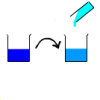Dilution Series Calculator

Age 14 to 16 Challenge Level:

Which dilutions can you make using 10ml pipettes and 100ml measuring cylinders?Drug Stabiliser

Age 16 to 18 Challenge Level:

How does the half-life of a drug affect the build up of medication in the body over time?Core Scientific Mathematics

Age 14 to 18 Challenge Level:

This is the area of the advanced stemNRICH site devoted to the core applied mathematics underlying the sciences.Why Multiply When You're about to Divide?

Age 16 to 18 Challenge Level:

A brief introduction to PCR and restriction mapping, with relevant calculations...Chemnrich

Age 14 to 18 Challenge Level:

chemNRICH is the area of the stemNRICH site devoted to the mathematics underlying the study of chemistry, designed to help develop the mathematics required to get the most from your study. . . .Robot Camera

Age 14 to 16 Challenge Level:

Could nanotechnology be used to see if an artery is blocked? Or is this just science fiction?Population Dynamics - Part 5

Age 16 to 18 Challenge Level:

Fifth in our series of problems on population dynamics for advanced students.Bird-brained

Age 16 to 18 Challenge Level:

How many eggs should a bird lay to maximise the number of chicks that will hatch? An introduction to optimisation.Ratios and Dilutions

Age 14 to 16 Challenge Level:

Scientists often require solutions which are diluted to a particular concentration. In this problem, you can explore the mathematics of simple dilutionsGenetic Intrigue

Age 16 to 18

Dip your toe into the fascinating topic of genetics. From Mendel's theories to some cutting edge experimental techniques, this article gives an insight into some of the processes underlying. . . .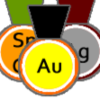Alternative Record Book

Age 14 to 18 Challenge Level:

In which Olympic event does a human travel fastest? Decide which events to include in your Alternative Record Book.Population Dynamics

Age 16 to 18 Challenge Level:

This problem opens a major sequence of activities on the mathematics of population dynamics for advanced students.Branching Processes and Extinction

Age 16 to 18 Challenge Level:

An advanced mathematical exploration supporting our series of articles on population dynamics for advanced students.Population Dynamics - Part 1

Age 16 to 18 Challenge Level:

First in our series of problems on population dynamics for advanced students.Population Dynamics - Part 2

Age 16 to 18 Challenge Level:

Second in our series of problems on population dynamics for advanced students.Population Dynamics - Part 3

Age 16 to 18 Challenge Level:

Third in our series of problems on population dynamics for advanced students.Population Dynamics - Part 4

Age 16 to 18 Challenge Level:

Fourth in our series of problems on population dynamics for advanced students.Population Dynamics - Part 6

Age 16 to 18 Challenge Level:

Sixth in our series of problems on population dynamics for advanced students.Population Ecology Using Probability

Age 16 to 18 Challenge Level:

An advanced mathematical exploration supporting our series of articles on population dynamics for advanced students.Population Dynamics Collection

Age 16 to 18 Challenge Level:

This is our collection of tasks on the mathematical theme of 'Population Dynamics' for advanced students and those interested in mathematical modelling.Predator - Prey Systems

Age 16 to 18 Challenge Level:

See how differential equations might be used to make a realistic model of a system containing predators and their prey.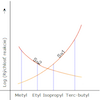Reaction Types

Age 16 to 18 Challenge Level:

Explore the rates of growth of the sorts of simple polynomials often used in mathematical modelling.Is Your DNA Unique?

Age 16 to 18 Challenge Level:

Use combinatoric probabilities to work out the probability that you are genetically unique!Exact Dilutions

Age 14 to 16 Challenge Level:

Which exact dilution ratios can you make using only 2 dilutions?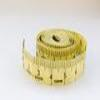Bigger or Smaller?

Age 14 to 16 Challenge Level:

When you change the units, do the numbers get bigger or smaller?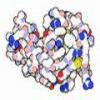Mixed up Mixture

Age 14 to 16 Challenge Level:

Can you fill in the mixed up numbers in this dilution calculation?Mathematical Issues for Biologists

Age 16 to 18 Challenge Level:

STEM students at university often encounter mathematical difficulties. This articles highlights the 8 key problems for biologists.Fitting Flat Shapes

Age 16 to 18 Challenge Level:

How efficiently can various flat shapes be fitted together?Packing 3D Shapes

Age 14 to 16 Challenge Level:

What 3D shapes occur in nature. How efficiently can you pack these shapes together?Catalyse That!

Age 16 to 18 Challenge Level:

Can you work out how to produce the right amount of chemical in a temperature-dependent reaction?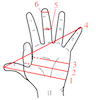Age 14 to 16 Challenge Level:

Which units would you choose best to fit these situations?Investigating the Dilution Series

Age 14 to 16 Challenge Level:

Which dilutions can you make using only 10ml pipettes?Mixing Ph

Age 16 to 18 Challenge Level:

Use the logarithm to work out these pH values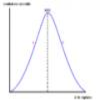Graphic Biology

Age 16 to 18 Challenge Level:

Several graphs of the sort occurring commonly in biology are given. How many processes can you map to each graph?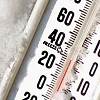Ph Temperature

Age 16 to 18 Challenge Level:

At what temperature is the pH of water exactly 7?Extreme Dissociation

Age 16 to 18 Challenge Level:

In this question we push the pH formula to its theoretical limits.Real-life Equations

Age 16 to 18 Challenge Level:

Here are several equations from real life. Can you work out which measurements are possible from each equation?Blood Buffers

Age 16 to 18 Challenge Level:

Investigate the mathematics behind blood buffers and derive the form of a titration curve.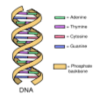The Genes of Gilgamesh

Age 14 to 16 Challenge Level:

Can you work out the parentage of the ancient hero Gilgamesh?A Question of Scale

Age 14 to 16 Challenge Level:

Use your skill and knowledge to place various scientific lengths in order of size. Can you judge the length of objects with sizes ranging from 1 Angstrom to 1 million km with no wrong attempts?Approximately Certain

Age 14 to 18 Challenge Level:

Estimate these curious quantities sufficiently accurately that you can rank them in order of sizeWhose Line Graph Is it Anyway?

Age 16 to 18 Challenge Level:

Which line graph, equations and physical processes go together?Bionrich

Age 14 to 18 Challenge Level:

bioNRICH is the area of the stemNRICH site devoted to the mathematics underlying the study of the biological sciences, designed to help develop the mathematics required to get the most from your. . . .Neural Nets

Age 16 to 18

Find out some of the mathematics behind neural networks.